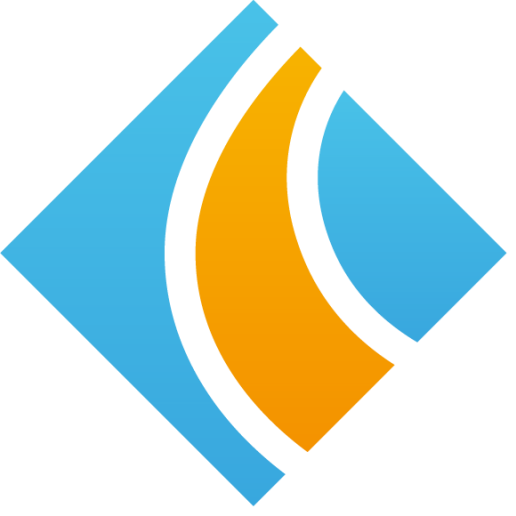Select Page

Objective Questions

1. Accounting standard FAS 133 applies to
a. Accounting for certain investments in debt and securities.
b. Accounting for derivative instruments and hedging activities.
c. Fair value measurements.
d. None of the above.

2. Premium paid towards purchase of call options purchased for speculative trade should be treated as
a. Expense.
b. Revenue.
c. Loss.
d. Gain.

3. For an options contract, the commission/brokerage paid is treated as follows:
a. Part of the cost of options contract.
b. Debited to commission/brokerage account.
c. Adjusted later in the broker account at the time of sale.
d. None of the above.

4. For ascertaining fair value at the end of the reporting period, the value of the entire position will be written back to the
a. Profit and loss account.
b. Expense account.
c. Income account.
d. None of the above.

5. Reversal of marked-to-market entry will happen on
b. Next valuation day.
c. Same day itself.
d. None of the above.

6. For call options (bought), if the market value of the underlying asset is over the strike price of the contact, then the excess amount denotes
a. Profit.
b. Loss.
c. Breakeven.
d. None of the above.

7. When the options contract is expired, then the option premium paid denotes
a. Expense.
b. Income.
c. Gain.
d. None of the above.

8. Exercising of an options contract means
a. Creation of underlying asset.
b. Breaking the options contract.
c. Paying the margin amount.
d. None of the above.

9. Buyback of the call options results in
a. Creating a fresh contract.
b. Squaring up of the existing short position.
c. Creating a long position.
d. None of the above.

10. Account treatment for a call options contract is decided based on
a. Position of the contract.
b. Intention of the purchase.
c. Size of the contract.
d. None of the above.

11. Assume that a call option with a quantity of 10,000 shares and a premium of \$6,000 has a value of \$9 at the end of the reporting period. Which of the following amounts is recognized as income which reflects as an asset on the balance sheet?
a. \$9,000
b. \$96,000
c. \$84,000
d. \$90,000
e. \$9,600

12. The accounting entry for mark-to-market reversal of a call option is not required if the valuation is done using the
a. Reversal method at the end of reporting period.
b. Black-Scholes model.
c. Incremental value method.
d. End of period market price.
e. Beginning of period market price.

13. When the call options are exercised, the investor has to pay for the underlying at
a. The current market price of the stock.
b. The rate at which the exchange decides.
c. The contracted strike price.
d. The previous day’s closing price.
e. None of the above.

14. If an investor writes a call option, then the investor ______________on such writing of the call option.
a. Would pay the premium on the exercise date.
b. Would collect the premium on the exercise date.
c. Would pay the premium on the settlement date.
d. Would collect the premium on the settlement date.
e. Would neither collect nor pay any premium.

15. When a call margin is initiated the investor would be required to pay an amount equal to ______ of the value of the position in addition to the potential loss as of that date.
a. 8 percent.
b. 15 percent.
c. 25 percent.
d. 12 percent.
e. 10 percent.